# GDB-9 Database

Database of molecular quantum calculations

Computed geometric, energetic, electronic, and thermodynamic properties for 134k stable small organic molecules made up of carbon, hydrogen, nitrogen, oxygen, and fluorine

## Examples

### Basic Examples

Retrieve the resource:

 In:=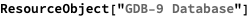Out=Retrieve the default content:

 In:=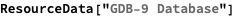Out=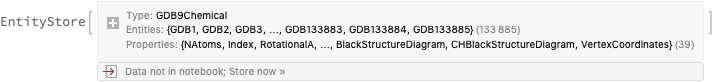Register the EntityStore:

 In:=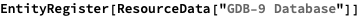Out=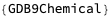### Analysis

Find the total number of entities:

 In:=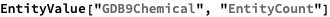Out=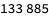Get the available properties for the entity store:

 In:=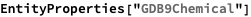Out=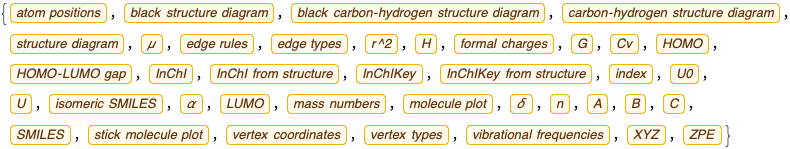View the property descriptions in a Dataset:

 In:=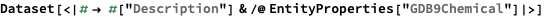Out=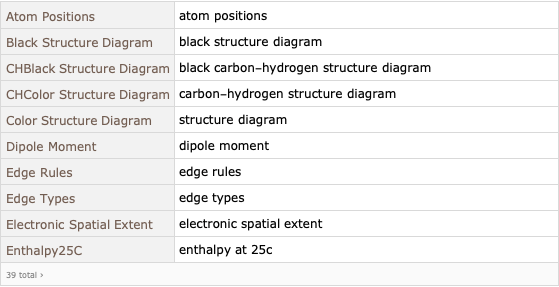Quickly view the properties of a random entity:

 In:=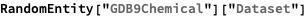Out=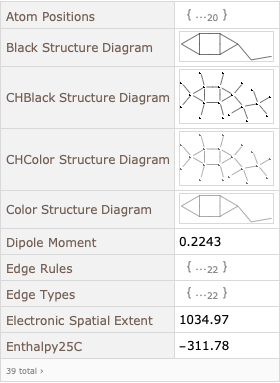### Visualization

Make a scatter plot comparing the internal energy calculated at absolute zero versus the HOMO-LUMO gap:

 In:=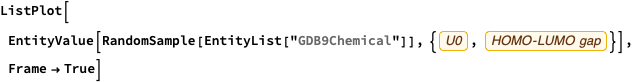Out=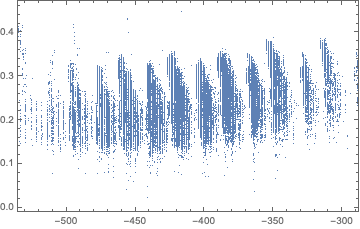In:=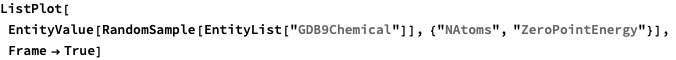Out=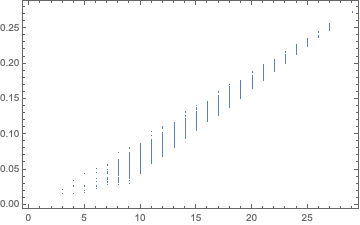Use the Wolfram Language Graph functionality to find the number of distinct rings in each molecule, then display this in a histogram:

 In:=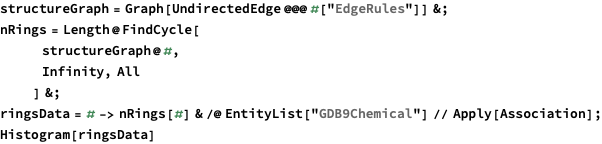Out=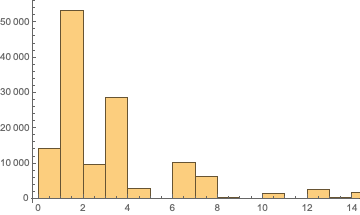Some of the molecules have as many as 14 rings! Look at their 3D structure:

 In:=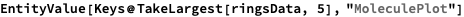Out=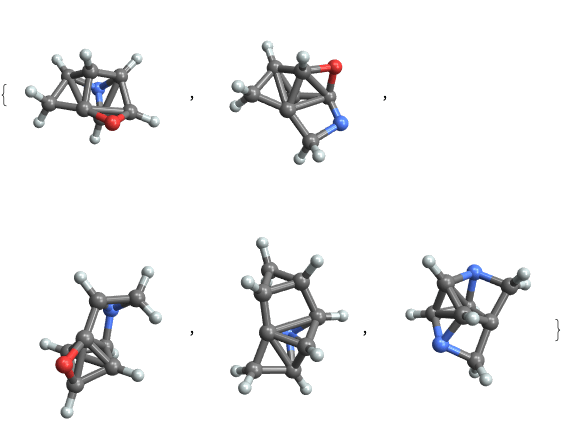Make a histogram from the maximum graph-distances:

 In:=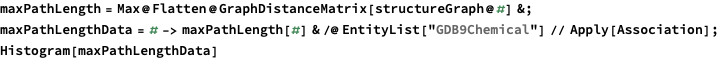Out=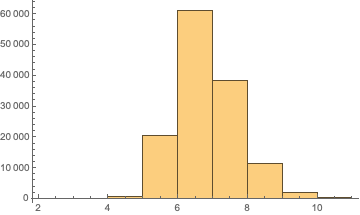Connect to PubChem to find all the names for a given entity (if it is in their database), using the SMILES string as a search parameter:

 In:=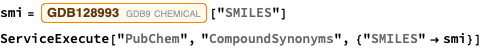Out=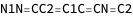Out=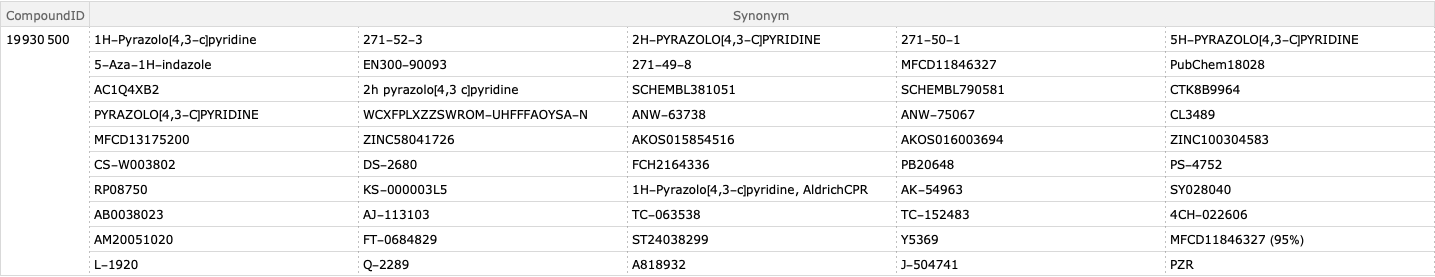Wolfram Research, "GDB-9 Database" from the Wolfram Data Repository (2017) https://doi.org/10.24097/wolfram.99752.data